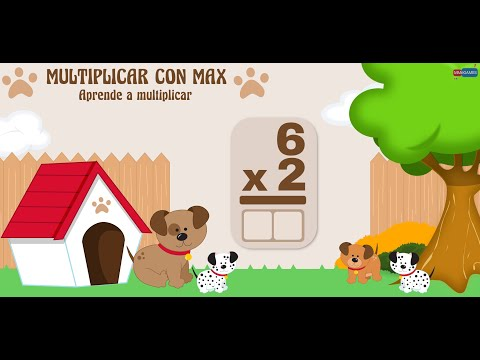### 7 apps and games to learn multiplication tables## With these apps your children will learn to multiply while having fun.

The mathematics can represent a challenge for some children, and if we talk about learning the multiplication tables things get a little more complicated.

Fortunately we have the help of technology, which can be very useful in these cases. There are very practical applications specially designed for children and to help them in the process of learn multiplication tables in a fun and practical way.Within the Google store we can find countless applications designed to learn mathematics. Some very well recommended by users.

Today we are going to present you a selection of the best and we are going to briefly explain what each one is about.

• Multiply with Max
• Math: multiplication
• Multiplication table: learn easily
• Learn to multiply
• Learn multiplication table
• Math games, Math
• Multiplication tables - free game

## Multiply with MaxThis is a free application designed so that children can easily learn the different multiplication tables through games.

Max is a friendly little dog who interacts with the children during all the games and exercises that the application includes and helps them achieve their goals.

The Multiply with Max app includes mini-games and you can select with which Multiplication table you want to practice. As you progress you can perform more difficult exercises.

The application includes time trials, exercises to practice and games to play with other players. A quick workout is included before participating in each “player versus player” challenge.

## Math: MultiplicationLearn the multiplication tables with exercises and training adapted to your level of study.

This app teaches how to do a multiplication through exercises. Use an intelligent method to understand your mistakes and to give yourself the proper guidance.

You can choose two methods to learn to multiply: the method learning and the method of training. With the learning method the application shows how to do a multiplication step by step giving a detailed explanation of each one.

With the training method, each one does the multiplication step by step and the application detects errors during the exercise and gives the necessary indications.

You can choose different levels of difficulty. The application calculates a score of time and errors during the exercises.

Mathematics: Multiplication is a free app and is available on Google play.

## Multiplication tableMultiplication Table includes “puzzle” type games to help you memorize the multiplication tables.

Multiplication table is another educational application that we can download for free designed to help children to learn multiplication tables easily while having fun.

The application is useful for all ages, from children to students of higher levels.

It includes several “puzzle” -type games to help train the brain to quickly solve math tests at both the basic and higher levels or prepare for tests.

## Learn to multiplyHelp Henry find the block with the correct multiplication result and level up.

Learn to Multiply is a free application with games with which you can learn the multiplication tables from 1 to 12.

Henry is a nice character that you have to help select the block with the correct answer. The application has a style similar to that of video games, where the character moves from right to left or jumping to reach the block with the correct answer to the multiplication. When you manage to solve 5 multiplications Without depleting the "lives" of the game you can level up.

The game has 4 levels in which the difficulty of the calculations to be solved is increased, it also includes a review section with the multiplication tables from 1 to 12.

## Learn multiplication tableLearn the multiplication tables while you solve the calculations to get food for the panda bear.

In this free application a cute little panda helps you learn multiplication tables while playing a fun math game.

Learn multiplied table is designed for younger children and was created with the help of teachers. The application uses the machine learning mechanism reducing the number of exercises that are easily solved and increasing the exercises that are more difficult to solve to allow learning through repetition.

During the game, the children must feed the panda bear and solve exercises to get more food.

You can choose the levels of difficulty and set the type of exercises - multiplication or division -.

## Math games, MathLearn the multiplication tables through math tricks, multiplication tables and games

Math games is a free application that includes math tricks, multiplication tables and math games with addition, subtraction, multiplication and division operations.

Besides games there are lessons and exercises for practice multiplication tables, math for kids, math tests and solving equations.

You can choose the level of complexity according to age and has 16 different types of math homework. It includes a grading system with graphs of results and new lessons are included with each update.

## Multiplication tablesThis app contains quiz-type games, player-versus-player duels and a school exam simulator so you can learn all the multiplication tables.

Times tables is a free game application for learn all tables from 1 to 10.

This application is designed for children, youth or adults and helps learn the tables progressively.

It includes quiz-type games, player versus player duels, and a school exam simulator. Contains quizzes, quizzes, and exercises to improve skills and measure progress.

With the help of these applications it will no longer be a problem for your boy or girl to learn to multiply, they can do it in a fun and easy way.